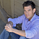4637 views
4637
```study("HullMA",overlay=true)
n=input(title="period",type=integer,defval=16)

n2ma=2*wma(close,round(n/2))
nma=wma(close,n)
diff=n2ma-nma
sqn=round(sqrt(n))

n2ma1=2*wma(close,round(n/2))
nma1=wma(close,n)
diff1=n2ma1-nma1
sqn1=round(sqrt(n))

n1=wma(diff,sqn)
n2=wma(diff1,sqn)
c=n1>n2?green:red
ma=plot(n1,color=c)

```Hey! Why does the hull ma change from red to green back to red. What moving average crosses are they to have them switch colors? I am trying to code a bot that buys when the hullma is green and sells when the hullma is red, and was wondering which moving averages cause them to change colors. Thanks!